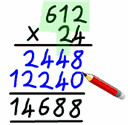# Long Multiplication

Long Multiplication is a special method for multiplying larger numbers.

It is a way to multiply numbers larger than 10 that only needs your knowledge of the ten times Multiplication Table.

Let us say we want to multiply

612 × 24

• First we multiply 612 × 4 (=2,448),
• then we multiply 612 × 20 (=12,240),
• and last we add them together (2,448 + 12,240 = 14,688).

But we can do better!

When we multiply 612 × 20 we only need to multiply 612 × 2 and place the result one column over (so it is the same as multiplying by 20).

We just have to be careful about lining up the columns. Here is how to do it (press the play button):

 We could also put a zero where the space is, like this:## More Than Two Digits

The same idea works when multiplying by more than two digit numbers. We just have to move over more spaces:

Have a try yourself with these Long Multiplication Worksheets